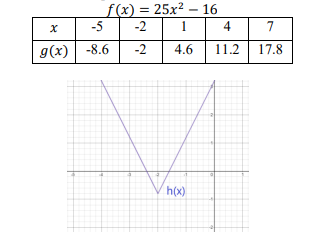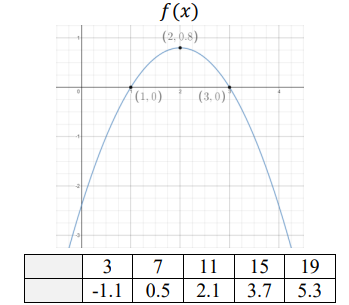# MA.912.F.1.6Export Print
Compare key features of linear and nonlinear functions each represented algebraically, graphically, in tables or written descriptions.

### Clarifications

Clarification 1: Key features are limited to domain; range; intercepts; intervals where the function is increasing, decreasing, positive or negative; end behavior and asymptotes.

Clarification 2: Within the Algebra 1 course, functions other than linear, quadratic or exponential must be represented graphically.

Clarification 3: Within the Algebra 1 course, instruction includes verifying that a quantity increasing exponentially eventually exceeds a quantity increasing linearly or quadratically.

General Information
Subject Area: Mathematics (B.E.S.T.)
Strand: Functions
Status: State Board Approved

## Benchmark Instructional Guide

• Domain
• Intercept
• Range
• Slope

### Vertical Alignment

Previous Benchmarks

Next Benchmarks

### Purpose and Instructional Strategies

In grade 8, students interpreted the slope and $y$-intercept of a linear equation in two variables. In Algebra I, students compare key features of two or more linear or nonlinear functions. Except for quadratic and exponential functions, nonlinear functions must be represented graphically. In later courses, students will compare key features of nonlinear functions represented graphically, algebraically, or with written descriptions.
• Within this benchmark, one of the functions given must be linear and the number of functions being compared is not limited to two.
• Problem types include comparing linear to nonlinear functions represented graphically and also opportunities that present linear, quadratic and exponential functions in different forms.
• Instruction includes student exploration of linear, quadratic, and exponential models to ultimately determine that a quantity increasing exponentially eventually exceeds a quantity increasing linearly or quadratically.
• For example, provide the following context.
• You are being contracted by a large company to provide technical services to a major engineering project. The contract will involve you advising a group of engineers for the three weeks. The company offers you a choice of two methods of payment for your services. The first is to receive \$500 per day of work. The second is to receive payment on a scale: two cents for one total day of work, four cents for two total days, eight cents for three total days, etc. Which method of payment would you choose?
• Have students choose a method of payment and begin a class discussion regarding the reasoning students used to make their choices (MTR.4.1). Ask if students can create a function to represent each payment method (MTR.7.1).
• Instruction includes representing domain and range using words, inequality notation and set-builder notation.
• Words
• If the domain is all real numbers, it can be written as “all real numbers” or “any value of $x$, such that $x$ is a real number.”
• Inequality notation
• If the domain is all values of $x$ greater than 2, it can be represented as $x$ > 2.
• Set-builder notation
• If the domain is all values of $x$ less than or equal to zero, it can be represented as {$x$|$x$ ≤ 0} and is read as “all values of $x$ such that $x$ is less than or equal to zero.”

### Common Misconceptions or Errors

• When describing domain or range, students may assign their constraints to the incorrect variable. In these cases, ask reflective questions to help students examine the meaning of the domain and range in the problem.
• Students may also miss the need for compound inequalities when describing domain or range. In these cases, use a graph of the function to point out areas of their constraint that would not make sense in context.
• When describing intervals where functions are increasing, decreasing, positive or negative, students may represent their interval using the incorrect variable.

### Strategies to Support Tiered Instruction

• Where students are struggling with concepts such as when a function is increasing, decreasing, positive, negative or questions about its end behavior, ask reflective questions:
• Imagine walking on the graph from left to right, where would you be going uphill (increasing) or where would you be going downhill (decreasing)?
• On the left, where are you coming from (far below or far above), and on the right, would you eventually be going up forever or down forever (end behavior)?

• Nancy works for a company that offers two types of savings plans. Plan A is represented by the function $g$($x$) = 250 + 3$x$, where $x$ is the number of quarter years she has utilized the plan. Plan B is represented by the function ($x$) = 250(1.01)$x$ , where $x$ is the number of quarter years she has utilized the plan.
• Part A. Nancy wants to have the highest savings possible after five years, when she plans to leave the company. Which plan should she use?
• Part B. What if Nancy stays for ten years? Which plan should she use?

• Three functions are represented below, with the table representing a linear function. Which function has the smallest $x$-intercept?### Instructional Items

Instructional Item 1
• The functions $f$($x$) and $g$($x$) are shown below, with $g$($x$) representing a linear function. Which function has the greater $y$-intercept?*The strategies, tasks and items included in the B1G-M are examples and should not be considered comprehensive.

## Related Courses

This benchmark is part of these courses.
1200310: Algebra 1 (Specifically in versions: 2014 - 2015, 2015 - 2022, 2022 and beyond (current))
1200320: Algebra 1 Honors (Specifically in versions: 2014 - 2015, 2015 - 2022, 2022 and beyond (current))
1200380: Algebra 1-B (Specifically in versions: 2014 - 2015, 2015 - 2022, 2022 and beyond (current))
1200400: Foundational Skills in Mathematics 9-12 (Specifically in versions: 2014 - 2015, 2015 - 2022, 2022 and beyond (current))
7912070: Access Mathematics for Liberal Arts (Specifically in versions: 2014 - 2015, 2015 - 2018, 2018 - 2019, 2019 - 2022, 2022 - 2023, 2023 and beyond (current))
7912090: Access Algebra 1B (Specifically in versions: 2014 - 2015, 2015 - 2018, 2018 - 2019, 2019 - 2022, 2022 and beyond (current))
1200315: Algebra 1 for Credit Recovery (Specifically in versions: 2014 - 2015, 2015 - 2022, 2022 and beyond (current))
1200385: Algebra 1-B for Credit Recovery (Specifically in versions: 2014 - 2015, 2015 - 2022, 2022 and beyond (current))
7912075: Access Algebra 1 (Specifically in versions: 2014 - 2015, 2015 - 2018, 2018 - 2019, 2019 - 2022, 2022 and beyond (current))
1207350: Mathematics for College Liberal Arts (Specifically in versions: 2022 and beyond (current))
1200710: Mathematics for College Algebra (Specifically in versions: 2022 and beyond (current))

## Related Access Points

Alternate version of this benchmark for students with significant cognitive disabilities.
MA.912.F.1.AP.6: Identify key features of linear, quadratic or exponential functions each represented in a different way algebraically or graphically (key features are limited to domain; range; intercepts; intervals where the function is increasing, decreasing, positive or negative; end behavior).

## Related Resources

Vetted resources educators can use to teach the concepts and skills in this benchmark.

## Formative Assessments

Comparing Linear and Exponential Functions:

Students are given a linear function represented by an equation and an exponential function represented by a graph in a real-world context and are asked to compare the rates of change of the two functions.

Type: Formative Assessment

Nonlinear Functions:

Students are asked to provide an example of a nonlinear function and explain why it is nonlinear.

Type: Formative Assessment

Compare Linear and Exponential Functions:

Students are asked to compare a linear function and an exponential function in context.

Type: Formative Assessment

## Perspectives Video: Experts

Birdsong Series: Mathematically Modeling Birdsong:

Richard Bertram discusses his mathematical modeling contribution to the Birdsong project that helps the progress of neuron and ion channel research.

Type: Perspectives Video: Expert

Birdsong Series: STEM Team Collaboration :

Researchers Frank Johnson, Richard Bertram, Wei Wu, and Rick Hyson explore the necessity of scientific and mathematical collaboration in modern neuroscience, as it relates to their NSF research on birdsong.

Type: Perspectives Video: Expert

## MFAS Formative Assessments

Compare Linear and Exponential Functions:

Students are asked to compare a linear function and an exponential function in context.

Comparing Linear and Exponential Functions:

Students are given a linear function represented by an equation and an exponential function represented by a graph in a real-world context and are asked to compare the rates of change of the two functions.

Nonlinear Functions:

Students are asked to provide an example of a nonlinear function and explain why it is nonlinear.

## Student Resources

Vetted resources students can use to learn the concepts and skills in this benchmark.

## Parent Resources

Vetted resources caregivers can use to help students learn the concepts and skills in this benchmark.